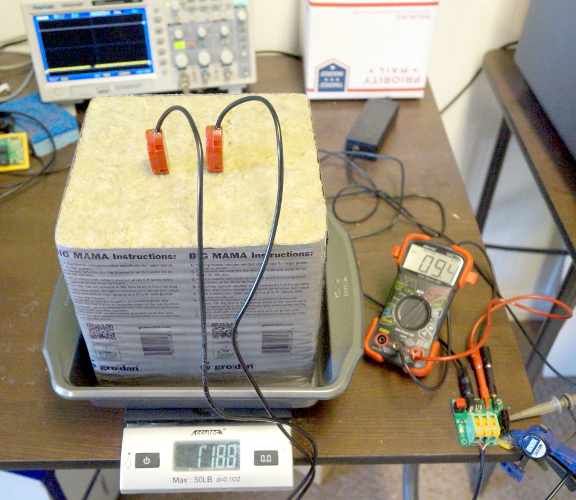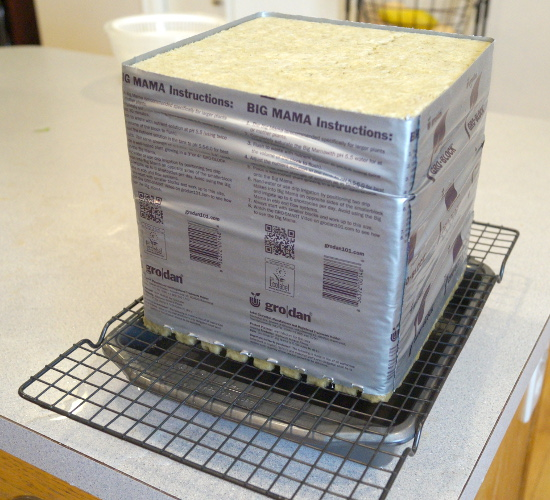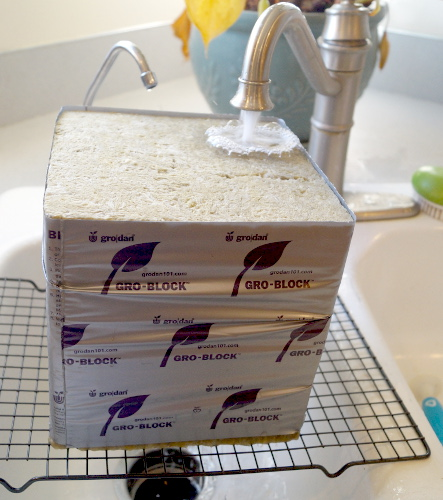# How to Measure Holding Capacity of Soil - TutorialHolding capacity of soil is the percentage of water that a given water can hold without dripping, after being saturated.

Sand has a low holding capacity, because water tends to drain out of it very easily. This is because the particle surface area density is less as particle size increases and so water tension decreases. Comparably, the holding capacity of clay soils is greater because the particle size is very small, and the water binds to the particles.

The picture above shows our setup. We are also correlating the VWC with readings from our VH400 moisture sensors.

## ToolsIt's very easy to measure holding capacity. You need the following items:

• A mailing scale set to grams,
• A container with holes in the bottom so water can drip out, or a in the case of rockwool a baking rack. It helps to choose a container where it easy to determine it's volume

## ProcedureIn our pictures in this tutorial we're using an 8 inch cube of rockwool as our soil.

• Dry the soil in an oven at a low temperature until it is completely dry.
• Weigh the container by itself, and note the weight: Mc.
• Figure out the volume of the container, and note the volume: Vc. If you have an irregular container. Plug the holes and fill with water and weigh it in grams. Each gram is 1ml of volume.
• Fill the container with the dry soil. If you fill it to the top even when saturated, then you can use the container's volume as the total volume Vt (Vt=Vc)
• Weigh the container with the dry soil, and note the weight: Ms.
• Weigh the container and dry soil, and note the weight.
• Saturate the soil with water.
• Wait until the soil stops dripping. When it stops dripping it is at the holding capacity.
• Weigh the wet soil, and note the weight: Mt.
• Compute the holding capacity in % VWC as calculated below.

## Calculations

Note that grams of water is the same as mililiters of water, so you can use them interchangibly.

Mw= Mt-Ms;

Where Mw is the mass of the water in grams.
Mt is the total mass of the continer and wet soil in grams.
Ms is the total mass of the continer and dry soil in grams.
Note that grams of water is the same as mililiters of water, so you can use them interchangibly.

Vw= Mw.

Holding_Capacity(VWC%) = Vw/Vt*100;

Where Vw is the volume of the water.
Where Vt is the total volume of the saturated soil. If you've filled the container to the top with saturated soil then this is the volume of the container.

## Results

For our 8 inch rockwool cube we calculated a holding capacity of 45%. It's our experience that most soils have a holding capacity of less that 50%.